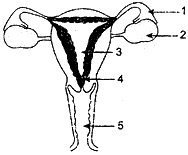Thursday , June 30 2022# 10 CBSE Board Science Paper 2018-19

## 10 CBSE Board Science Paper 2018-19

Time allowed: 3 hours
Date: 13/03/2019
M.M.: 80
Class: 10th
Subject: Science

## General Instructions:

• The question paper comprises five sections, A, B, C, D and E. You are to attempt all the sections.
• All questions are compulsory.
• Internal choice is given in sections B, C, D and E.
• Question numbers 1 and 2 in Section A are marks questions. They are to answered in one word or in one sentence.
• Question number 3 to 5 in Section B are two-marks questions. These are to be answered in about 30 words each.
• Question number 6 to 15 in Section C are three-marks questions. These are to be answered in about 50 words each.
• Question number 16 to 21 in Section D are five-marks questions. These are to be answered in about 30 words each.
• Question number 22 to 27 in Section E are based on practical skills. Each question is a two marks question. These are to be answered in brief.

## Section D

#### (a) the total resistance of the circuit, (b) the current through the circuit, (c) the potential difference across the (i) electric lamp and (ii) conductor, and (d) power of the lamp.#### (a) Identify the given diagram. Name the parts 1 to 5.## 11th Class Physics Term 2 Question Paper 2021-22

School Name: Himalaya Public School, Sector 13, Rohini, Delhi 110085 India Class: 11th Standard (CBSE) …